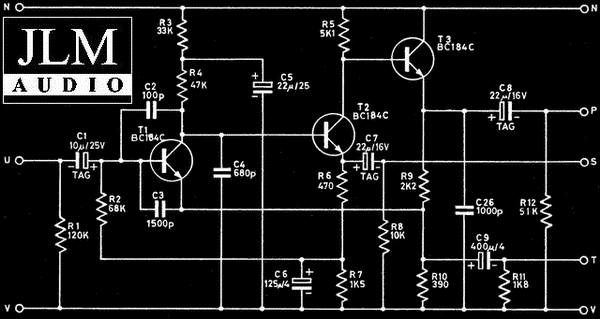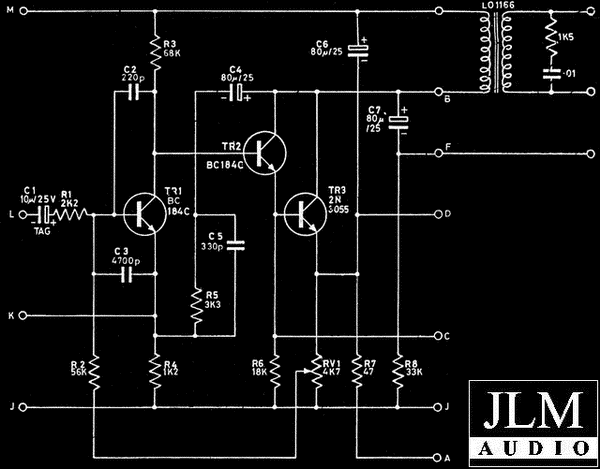Neve BA283 preamp section variationsOriginal Neve Pre Amp Component Variations to this circuit C1 = 6.8uF or 10uF or 22uF Tag Tantalum Capacitor C2 = 100pF Polystyrene Capacitor C3 = 1000pF or 1200pF or 1500pF Polystyrene Capacitor C4 = 680pF Polystyrene Capacitor C5 = 22uF 25v to 63v Electro Capacitor C6 = 125uF  to 150uF 4v Electro Capacitor C7 = 22uF 16v Tag Tantalum Capacitor C8 = 22uF 16v Tag Tantalum Capacitor C9 = 400uF 4v Electro Capacitor C26 = 1000pF Polystyrene Capacitor T1 = BC109 or BC184C picked for gain higher than 600 to minimise input noise (BC109 = BC549C) T2 = BC107 or BC184C (BC107 = BC547) T3 = BC107 or BC184C (BC107 = BC547) Neve BA283 Output Stage sectionOriginal Neve Output Component Variations to this circuit C1 = 10uF or 22uF Tag Tantalum Capacitor C2 = 220pF Polystyrene Capacitor C3 = 4700pF Polystyrene Capacitor C4 = 80uF or 100uF 25v Electro Capacitor C5 = 330pF Polystyrene Capacitor C6 = 80uF or 100uF 25v Electro Capacitor C7 = 80uF or 100uF 25v Electro Capacitor T1 = BC109 or BC184C picked for gain higher than 600 to minimise input noise (BC109 =  BC549C) T2 = BC107 or BC184C (BC107 =  BC547) T3 = 2N3055